Monday 01st June 2020
 CBSE Guess > Papers > Question Papers > Class XII > 2005 > Physics > Delhi Set-II PHYSICS 2005 (Set II—Delhi)
Except for the following questions all the remaining questions have been asked in Set I..  Q. 1. Why does the electric field inside a dielectric decrease when it is placed in an external electric field? 1 Q. 2. A charged particle enters into a uniform magnetic field and experiences an upward force as indicated in the figure. What is the charge sign on the particle? 1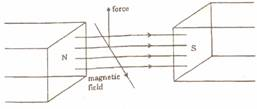Q. 4. Name the types of communication systems according to the mode of the transmission. 1 Q. 8. The figure shows two identical rectangular loops (1) and (2), placed on a table along with a straight long current carrying conductor between them. (i) What will be the directions of the induced currents in the loops when they are pulled away from the conductor with same velocity U? (ii) Will the e.m.f. induced in the two loops be equal? Justify your answer.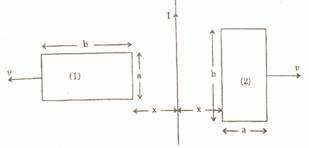Q. 10. A voltage of 30 V is applied across a carbon resistor with first, second and third rings of blue, black and yellow colours respectively. Find the value of cu- rent through the resistor. 2 Q. 11. Two dielectric slabs of dielectric constants K1 and K2 are filled in between the two plates, each of area A, of the parallel plate capacitor as shown in the figure. Find the net capacitance of the capacitor. 2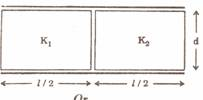Or A small metal sphere carrying charge +Q is located at the centre of a spherical cavity in a large uncharged metal sphere as shown in the figure. Use Gauss' theorem to find electric field at points P 1 and P 2 .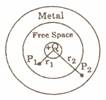Q. 17. A converging lens has a focal length of 20cm in air. It is made of material of refractive index 1.6.lf it is immersed in a liquid of refractive 1.3, what will be its new focal length? How does the nature of the lens change if this lens is immersed in a liquid of refractive index 1.8? 3 Q. 18. Explain the following terms: 3 (i) Ground waves (ii) Space waves (iii) Sky waves Q. 21. Explain, with the help of a circuit diagram, how the thickness of depletion layer in a p-n junction diode changes when it is forward biased. In the follow- ing circuits which one of the two diodes is forward biased and which is reverse biased? 3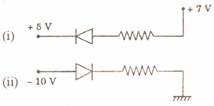Physics 2005 Question Papers Class XII Delhi Outside Delhi Compartment Delhi Compartment Outside Delhi Set I Set I Set I Set I Set II Set II Set II Set II Set III Set III Set III CBSE 2005 Question Papers Class XII